Courses

# RD Sharma Solutions for Class 8 Math Chapter 4 - Cubes and Cube Roots (Part-5) Class 8 Notes | EduRev

## RD Sharma Solutions for Class 8 Mathematics

Created by: Abhishek Kapoor

## Class 8 : RD Sharma Solutions for Class 8 Math Chapter 4 - Cubes and Cube Roots (Part-5) Class 8 Notes | EduRev

The document RD Sharma Solutions for Class 8 Math Chapter 4 - Cubes and Cube Roots (Part-5) Class 8 Notes | EduRev is a part of the Class 8 Course RD Sharma Solutions for Class 8 Mathematics.
All you need of Class 8 at this link: Class 8

#### Answer 1: Because 7 lies between 1 and 100, we will look at the row containing 7 in the column of x.By the cube root table, we have: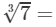1.913

#### Answer 2: Because 70 lies between 1 and 100, we will look at the row containing 70 in the column of x.By the cube root table, we have: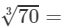4.121

#### Question 3: Making use of the cube root table, find the cube root 700

700=70×10700=70×10
Cube root of 700 will be in the column of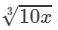against 70.

By the cube root table, we have: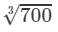=8.879

#### Question 4: Making use of the cube root table, find the cube root 7000

7000=70×1007000=70×100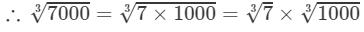By the cube root table, we have: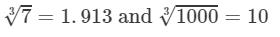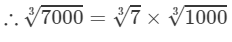=1.913×10=19.13

#### Question 5: Making use of the cube root table, find the cube root 1100

1100=11×1001100=11×100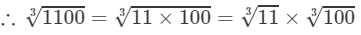By the cube root table, we have: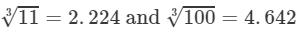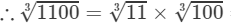=2.224×4.642=10.323 (Up to three decimal places)11003=113×1003=2.224×4.642=10.323 (Up to three decimal places)

#### Question 6: Making use of the cube root table, find the cube root 780

780=78×10780=78×10

Cube root of 780 would be in the column of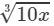against 78.

By the cube root table, we have: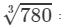= 9.205

#### Question 7: Making use of the cube root table, find the cube root 7800

7800=78×1007800=78×100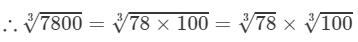By the cube root table, we have: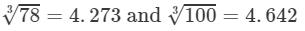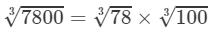=4.273×4.642=19.835 (upto three decimal places)

#### Answer 8: By prime factorisation, we have:

1346=2×673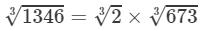Also

670<673<680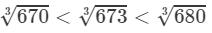From the cube root table, we have: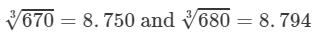For the difference (680-670), i.e., 10, the difference in the values

=8.7948.750=0.044=8.794-8.750=0.044

For the difference of (673-670), i.e., 3, the difference in the values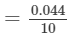×3=0.0132=0.013=0.04410×3=0.0132=0.013 (upto three decimal places)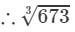=8.750+0.013=8.7636733=8.750+0.013=8.763

Now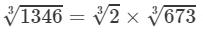=1.260×8.763=11.04113463=23×6733=1.260×8.763=11.041 (upto three decimal places)

#### Question 9: Making use of the cube root table, find the cube root 250

250=25×100250=25×100

Cube root of 250 would be in the column of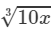against 25.

By the cube root table, we have: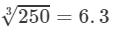Thus, the required cube root is 6.3.

#### Answer 10: By prime factorisation, we have:

5112=23×32×71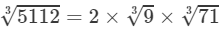By the cube root table, we have: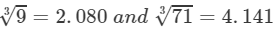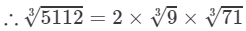=2×2.080×4.141=17.22751123=2×93×713=2×2.080×4.141=17.227 (upto three decimal places)

Thus, the required cube root is 17.227.

#### Question 11: Making use of the cube root table, find the cube root9800

9800=98×1009800=98×100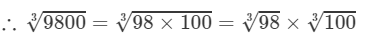By cube root table, we have: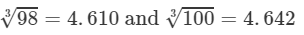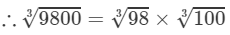=4.610×4.642=21.4098003=983×1003=4.610×4.642=21.40 (upto three decimal places)

Thus, the required cube root is 21.40.

#### Question 12: Making use of the cube root table, find the cube root 732

730<732<740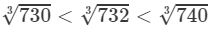From cube root table, we have: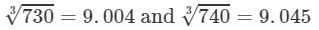For the difference (740-730), i.e., 10, the difference in values

=9.0459.004=0.041=9.045-9.004=0.041

For the difference of (732-730), i.e., 2, the difference in values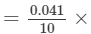2=0.0082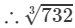=9.004+0.008=9.012

#### Question 13: Making use of the cube root table, find the cube root 7342

7300<7342<7400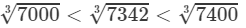From the cube root table, we have: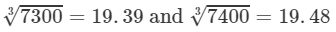For the difference (7400-7300), i.e., 100, the difference in values

=19.4819.39=0.09

For the difference of (7342-7300), i.e., 42, the difference in the values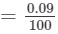×42=0.0378=0.037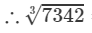=19.39+0.037=19.427

#### Question 14: Making use of the cube root table, find the cube root 133100

133100=1331×100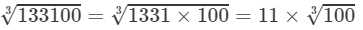By cube root table, we have: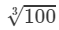=4.6421003=4.642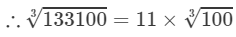=11×4.642=51.062

#### Question 15: Making use of the cube root table, find the cube root 37800

37800=23×33×175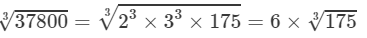Also

170<175<180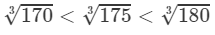From cube root table, we have: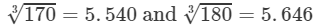For the difference (180-170), i.e., 10, the difference in values

=5.6465.540=0.106=5.646-5.540=0.106

For the difference of (175-170), i.e., 5, the difference in values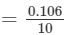×5=0.053=0.10610×5=0.053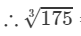=5.540+0.053=5.5931753=5.540+0.053=5.593

Now

37800=6×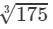=6×5.593=33.55837800=6×1753=6×5.593=33.558

Thus, the required cube root is 33.558.

#### Answer 16: The number 0.27 can be written as 27/100.

Now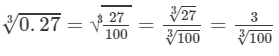By cube root table, we have: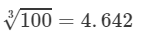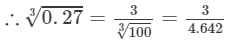=
0.646
0.273=31003=34.642=0.646
.

Thus, the required cube root is 0.646.

#### Answer 17: The number 8.6 can be written as 86/10.

Now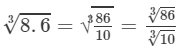By cube root table, we have: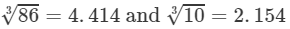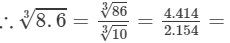2.0498.63=863103=4.4142.154=2.049

Thus, the required cube root is 2.049.

#### Answer 18: The number 0.86 could be written as 86/100.

Now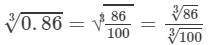By cube root table, we have: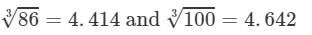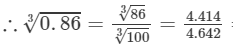=0.9510.863=8631003=4.4144.642=0.951 (upto three decimal places)

Thus, the required cube root is 0.951.

#### Answer 19: The number 8.65 could be written as 865/100.

Now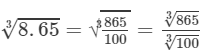Also

860<865<870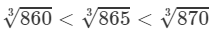From the cube root table, we have: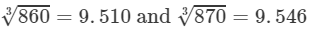For the difference (870-860), i.e., 10, the difference in values

=9.5469.510=0.036=9.546-9.510=0.036
For the difference of (865-860), i.e., 5, the difference in values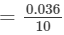×5=0.018  (upto three decimal places)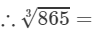9.510+0.018=9.5288653=9.510+0.018=9.528 (upto three decimal places)

From the cube root table, we also have: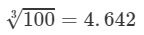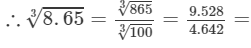2.0538.653=86531003=9.5284.642=2.053 (upto three decimal places)

Thus, the required cube root is 2.053.

#### Question 20: Making use of the cube root table, find the cube root 7532

7500<7532<7600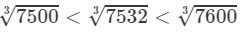From the cube root table, we have: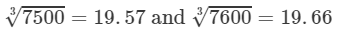For the difference (7600-7500), i.e., 100, the difference in values

=19.6619.57=0.09=19.66-19.57=0.09

For the difference of (7532-7500), i.e., 32, the difference in values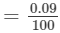×32=0.0288=0.029=0.09100×32=0.0288=0.029 (up to three decimal places)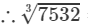=19.57+0.029=19.59975323=19.57+0.029=19.599

#### Question 21: Making use of the cube root table, find the cube root833

830<833<840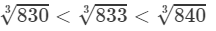From the cube root table, we have: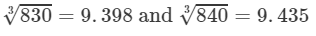For the difference (840-830), i.e., 10, the difference in values

=9.4359.398=0.037=9.435-9.398=0.037

For the difference (833-830), i.e., 3, the difference in values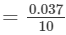×3=0.0111=0.011=0.03710×3=0.0111=0.011 (upto three decimal places)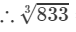=9.398+0.011=9.4098333=9.398+0.011=9.409

#### Answer 22: The number 34.2 could be written as 342/10.

Now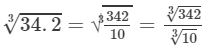Also

340<342<350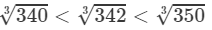From the cube root table, we have: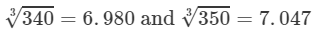For the difference (350-340), i.e., 10, the difference in values

=7.0476.980=0.067=7.047-6.980=0.067.

For the difference (342-340), i.e., 2, the difference in values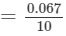×2=0.013=0.06710×2=0.013  (upto three decimal places)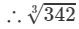=6.980+0.0134=6.9933423=6.980+0.0134=6.993 (upto three decimal places)

From the cube root table, we also have: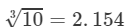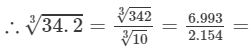3.246

Thus, the required cube root is 3.246.

#### Answer 23: Volume of a cube is given by:

V=a3V=a3, where a = side of the cube

∴∴ Side of a cube = a=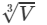If the volume of a cube is 275 cm3, the side of the cube will be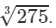We have:

270<275<280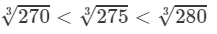From the cube root table, we have: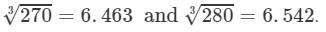For the difference (280-270), i.e., 10, the difference in values

=6.5426.463=0.079=6.542-6.463=0.079

For the difference (275-270), i.e., 5, the difference in values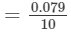×5=0.0395   0.04=0.07910×5=0.0395  ≃ 0.04 (upto three decimal places)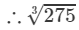=6.463+0.04=6.5032753=6.463+0.04=6.503 (upto three decimal places)

Thus, the length of the side of the cube is 6.503 cm.

91 docs

,

,

,

,

,

,

,

,

,

,

,

,

,

,

,

,

,

,

,

,

,

;